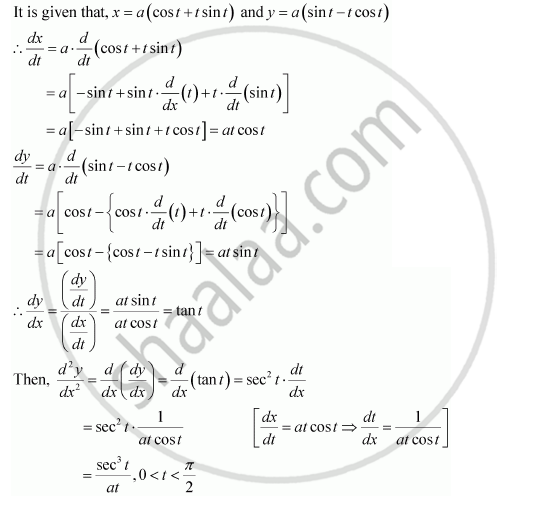# If X = a (Cos T + T Sin T) and Y = a (Sin T – T Cos T), Find (D^2y)/Dx^2 - Mathematics

If x = a (cos t + t sin t) and y = a (sin t – t cos t), find (d^2y)/dx^2

#### SolutionConcept: Logarithmic Differentiation
Is there an error in this question or solution?

#### APPEARS IN

NCERT Class 12 Maths
Chapter 5 Continuity and Differentiability
Q 17 | Page 192